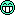## The unknown ρ [Power / Sample Size]

Hi Chris,

❝ ❝ Oh dear, that one! Opening Pandora’s box.

❝ And my apologies for opening the Pandora's box! I will pretend that I never wrote this❝ Here, I still am a bit confused. Since my alternative is T1=R AND T2=R, I need to carry out 2 tests. Both have to be rejected at a given significance (without adjustment, say 0.05) and jointly, these 2 tests have to achieve a given power (say 0.8). Here is where I am confused:

❝ 1) The 2 tests must jointly have a power > 0.8. For illustration, if we assume independence of these 2 tests (which is clearly not the case but for the sake of illustration), we want to achieve the following:

❝ power(test1, test2) = power(test1) x power(test2) = 0.8

❝ If we further assume equal power for test 1 and test 2, then we need sqrt(0.8) = 0.9 for each test. When computing the sample size in PowerTOST, I am wondering whether the power argument is for the joint tests, or for individual test. If it's specified at an individual level, the joint power is 0.8 x 0.8 = 0.64.

That’s a fallacy. I fell into this trap myself. See post dealing with overall power of multiple studies.

❝ 2) I understand that T1 and T2 are treated independently. But are they assumed to be independent from R, as well?

❝ Even if T1, T2 and R are pairwise independent, R appears in both test statistics so the two tests will show correlation.

Some, yes. To which degree is unknown. Consider this: AUC is the PK metric of extent of absorption and Cmax the one of rate of absorption. Where the former in comparative BA depends only on the fractions absorbed ƒ (we assume that the doses are identical and clearances are constant), the latter is a composite metric, i.e., depends on ƒ, ka, and ke. Hence, it is clear that they are correlated, but how much?

(Almost) everything is possible in PowerTOST.

library(PowerTOST) # CV and theta0 must be two-element vectors, values can be different # below identical ones for simplicity CV     <- c(0.20, 0.20) # assumed CVs theta0 <- c(0.95, 0.95) # assumed T/R-ratios target <- 0.80 design <- "2x2" rho    <- seq(0.5, 1, 0.1) res    <- data.frame(rho = rho, n = NA_integer_, power = NA_real_) for (j in seq_along(rho)) {   res[j, 2:3] <- sampleN.2TOST(CV = CV, theta0 = theta0, design = design,                                targetpower = target, rho = rho[j],                                print = FALSE)[7:8] } print(res, row.names = FALSE)  rho  n   power  0.5 24 0.82667  0.6 24 0.83335  0.7 22 0.80145  0.8 22 0.81385  0.9 22 0.82943  1.0 20 0.83606

Looks great on paper. The higher the correlation, the higher the power or the other way ’round, the lower the sample size for similar power.

❝ Does this correlation taken into account when computing the sample size?

❝ In my naive opinion, I feel that, under the alternative which stipulates BE, the PK parameters should be correlated between treatments and this correlation should be taken into account.

As you rightly wrote, since R occurs in both tests, likely there is some correlation indeed. But (but!) from where and how will you get it?
In the exercise of two metrics we failed to establish a consistent value (see this lenghty thread for our desperate attempts)… With T1=R AND T2=R we are fishing in the dark. You would have to perform a couple of studies (at least three) to come up with an estimate of $$\rho$$ which you could use in the sample size estimation of the fourth.Dif-tor heh smusma 🖖🏼 Довге життя Україна!Helmut SchützThe quality of responses received is directly proportional to the quality of the question asked. 🚮
Science QuotesIng. Helmut Schütz